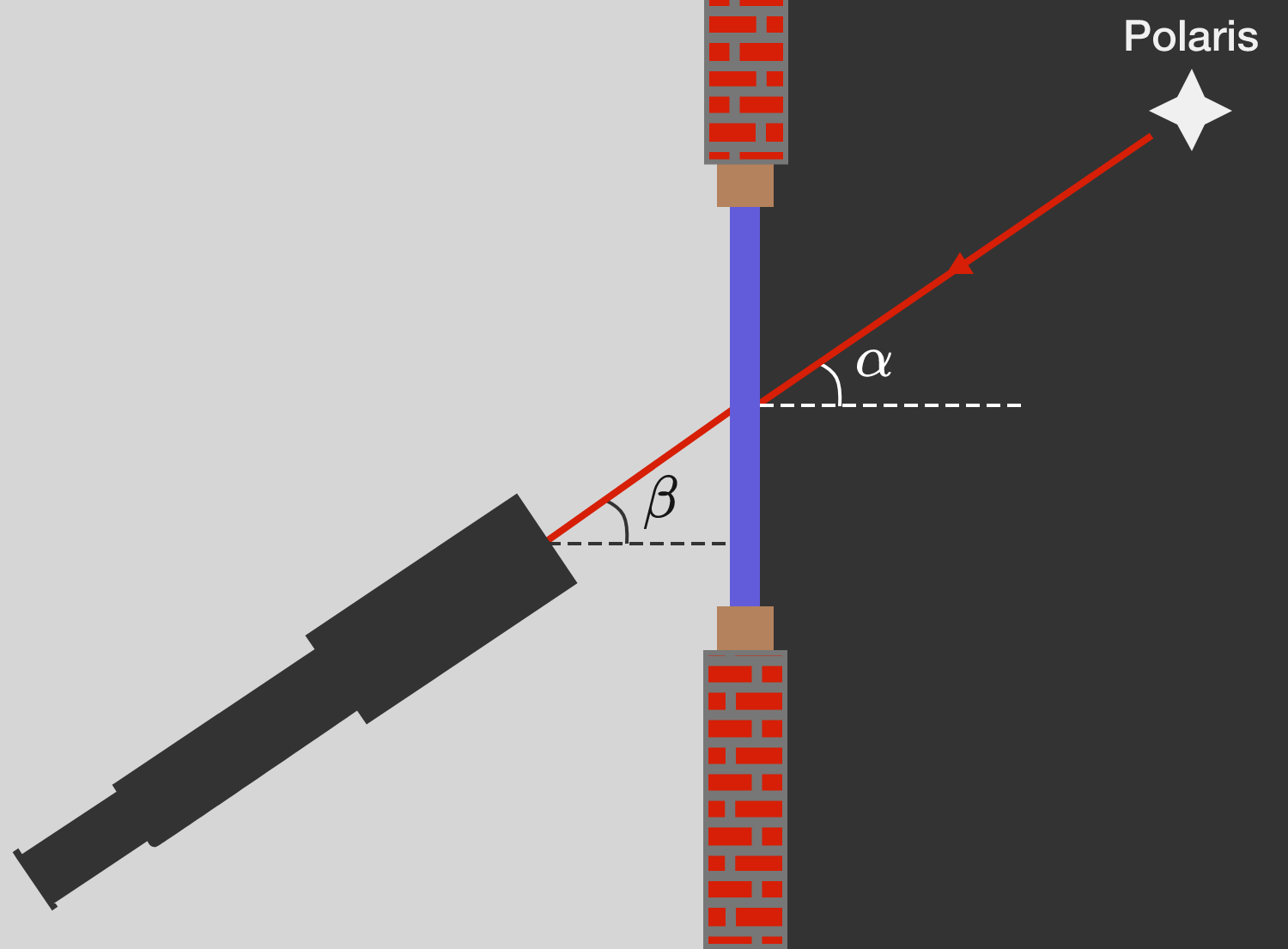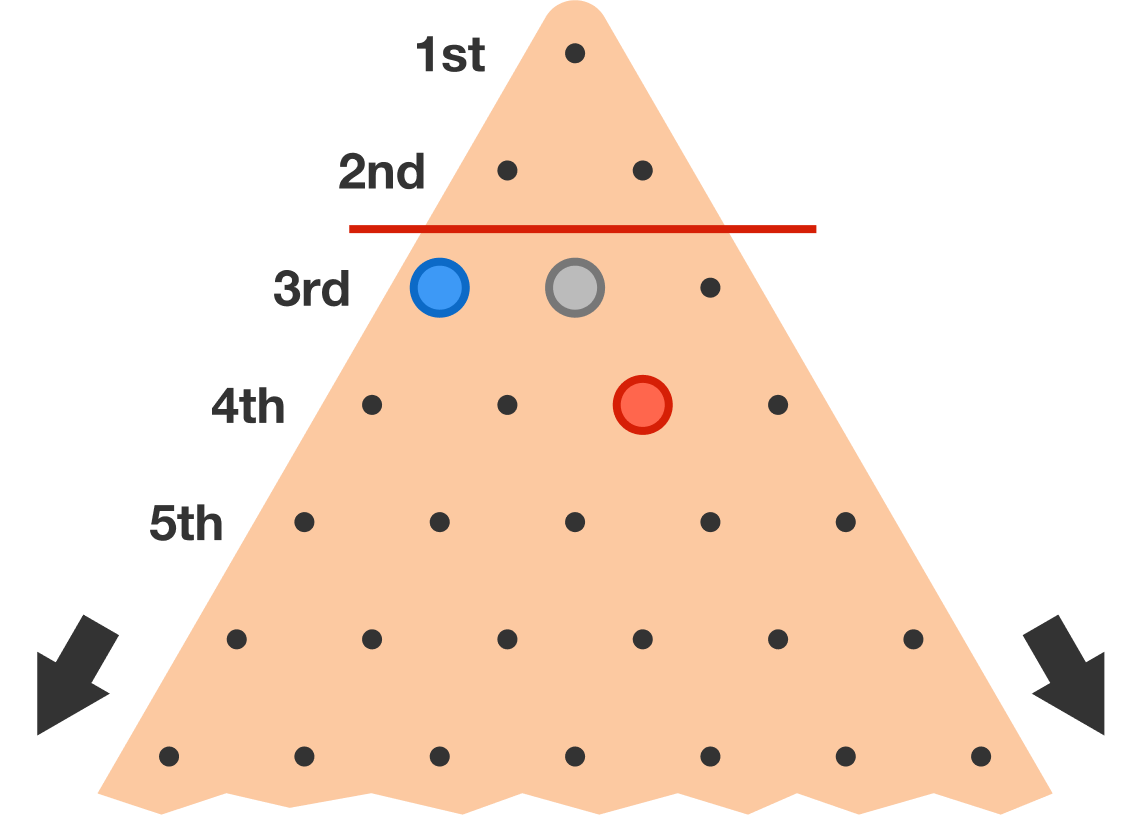# Problems of the Week

Contribute a problem

$\large f_n(x) = \Large \underbrace{x^{x^{ x^{x^{\cdot^{\cdot^{\cdot ^x}}}}}}}_{\text{ number of } x \text{'s = } n}$

What is $\displaystyle \lim_{x\to0^+} \big(f_{2016} (x) + f_{2017} (x) + f_{2018}(x)\big)?$

$S=\dfrac { 1 }{ 1\cdot 2\cdot 3 } +\dfrac { 1 }{ 3\cdot 4\cdot 5 } +\dfrac { 1 }{ 5\cdot 6\cdot 7 } +\dfrac { 1 }{ 7\cdot 8\cdot 9 } +\frac { 1 }{ 9\cdot 10\cdot 11 } +\cdots$

The sum $S$ can be expressed in the form $\ln { A } -\frac { 1 }{ A },$ where $A$ is a prime number.

What is $A?$

Galileo Gallei measured the angular positions of the stars with his telescope. To adjust his telescope, he initially aimed at the Pole Star, which is always at the same position in the sky at an angle of $\alpha$ with the horizontal.

On a cold winter's night of $\SI{-3}{\celsius},$ he conducted his measurements inside his study room at $\SI{20}{\celsius}.$ The starlight fell through his window and refracted twice on the glass. From his room, Polaris appeared at an angle of $\beta$ with the horizon.

What is the angle difference $\delta = \beta - \alpha$ between measurements inside and outside?Assumptions:

• Galileo's study room was in Venice, at a latitude of $\phi \approx \SI{45}{\degree}.$
• Air is an ideal gas with the specific gas constant $R_\text{air}^\ast \approx 300 \text{ J}/(\text{kg} \cdot \text{K}).$
• Air is a dielectric with an electric susceptibility of $\chi_\text{air} = \varepsilon_\text{air} - 1 = c \cdot \rho_\text{air}\, \big(\text{where } c \approx 10^{-3} \text{ m}^3/\text{kg}\big).$

Given that $y=f(x), \frac{dy}{dx}=x^{-4}+y^2,$ and $f\big(\frac{1}{\pi}\big)=-\pi,$ find $f\big(\frac{4}{3\pi}\big).$

In an infinitely large triangular peg board game, the goal is to get a peg into the top space on the board through a series of moves. A move consists of a peg jumping over an adjacent peg, which eliminates the peg which was jumped over.

It is possible to get a peg into the top space when all the starting pegs are below the $2^\text{nd}$ row. This starting orientation is shown below.

What is the largest possible $n$ such that we can get a peg into the top space when all the starting pegs are below the $n^\text{th}$ row?Red jumps Grey, then Blue jumps Red. This puts Blue into the top space.

×